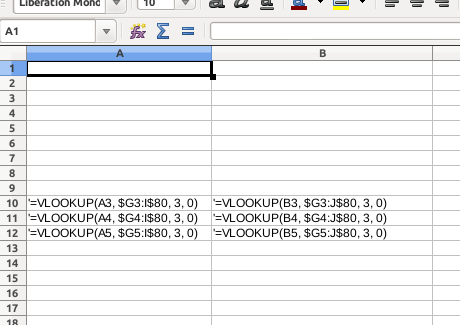# How to stop array reference changing when copying VLOOKUP formula?

Libreoffice calc 4.3.7.2 on Ubuntu 14.10

I have a VLOOKUP formula that when I copy and paste down the column, I get

``````=VLOOKUP(A3, G3:I80, 3, 0)
=VLOOKUP(A4, G4:I81, 3, 0)
=VLOOKUP(A5, G5:I82, 3, 0)
=VLOOKUP(A6, G6:I83, 3, 0)
``````

The array reference, the 2nd parameter, changes as I go down the column. I need the array reference to stay the same, like

``````=VLOOKUP(A3, G3:I80, 3, 0)
=VLOOKUP(A4, G3:I80, 3, 0)
=VLOOKUP(A5, G3:I80, 3, 0)
=VLOOKUP(A6, G3:I80, 3, 0)
``````

otherwise, obviously, the formula will be looking up to a different array of data in each cell.

How can this be done?

Hi mlpqlm

You can use a ‘`\$`’ (U+0024) DOLLAR SIGN to instruct Calc not to rewrite the references. Each cell name is in 2 parts (ignoring the Sheet name), so there are 4 options:-

• `G3` = rewrite both columns + rows on copy
• `\$G3` = keep the columns fixed on copy but allow the rows to be rewritten
• `G\$3` = allow the columns to be rewritten but keep the rows fixed on copy
• `\$G\$3` = fix both columns + rows on copy

Here’s a picture of it + this is what I did.

1. Placed `'=VLOOKUP(A3, \$G3:I\$80, 3, 0)`’ in A10
1. copied A10 to A10→B12
2. Placed a ’ (U+0027) APOSTROPHE at the beginning of each cell with an entryIf this helps then please tick the answer ()

…and/or show you like it with an uptick ()

+1 just what I needed.